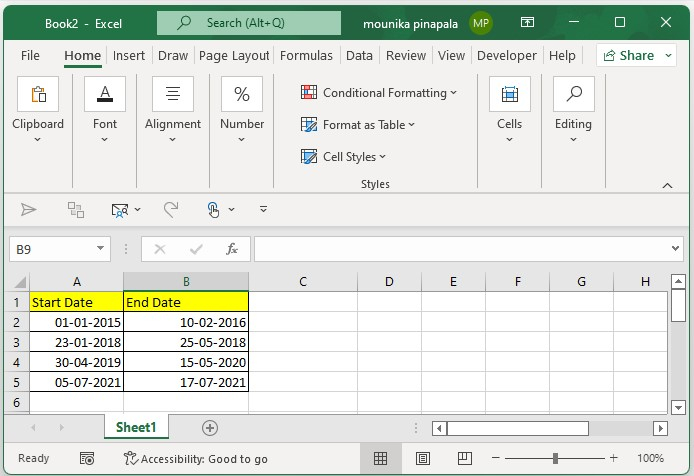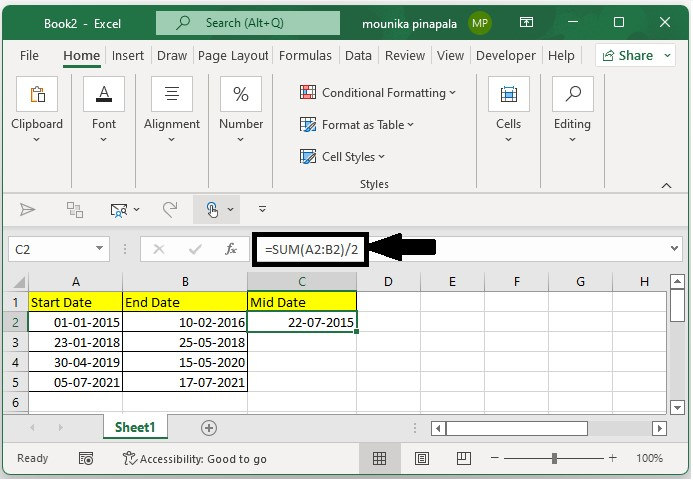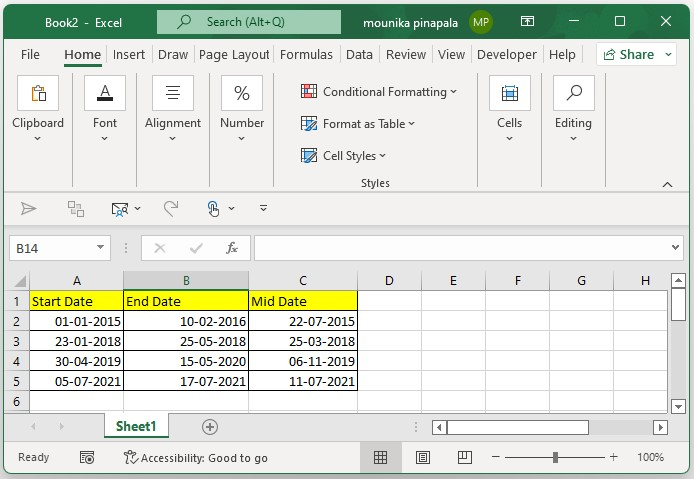# How to calculate the midpoint between two dates in Excel?

In this tutorial, we will use a simple example to demonstrate how you can use Excel to get the midpoint date between two given dates. Follow the steps given below.

## Step 1

Open a Microsoft Excel Sheet, and enter the two dates’ start date, and the end date as shown in the below screenshot for your reference. You can also change the dates as per your wish.## Step 2

Now you need to type the below given formula in a blank cell besides the start date and end date, and then press enter key as shown in the below screenshot for your reference.## Step 3

Now you need to drag the fill handle down to where you want to calculate the midpoint date to get the midpoint date between the start date and end date as shown in the below given screenshot for your reference.In the above screenshot, you will find the midpoint dates between two given dates (start date and end date)

Note − In the formula that we used, the Start date is mentioned in the cell A2 in a Microsoft Excel sheet the and end date is mentioned in B2 in a Microsoft excel sheet. You can change this start date and end date as per your requirement.

## Conclusion

There are also other methods to calculate the mid points between two given dates (start dates and end dates). However, what we have shown in this tutorial is the easiest and the most frequently used method to calculate the midpoint date from the given two dates (start date and end date) in a Microsoft excel sheet.

Updated on: 06-Feb-2023

328 Views